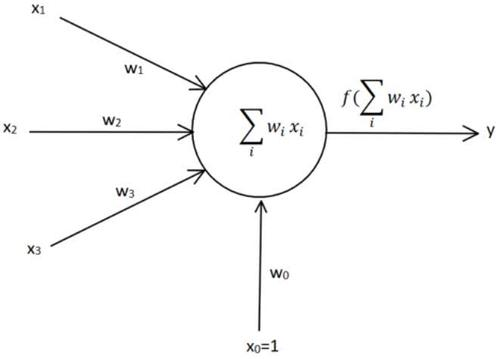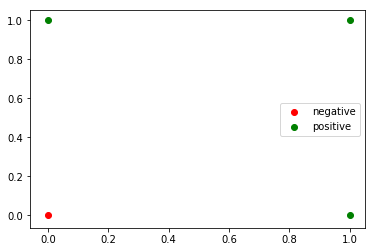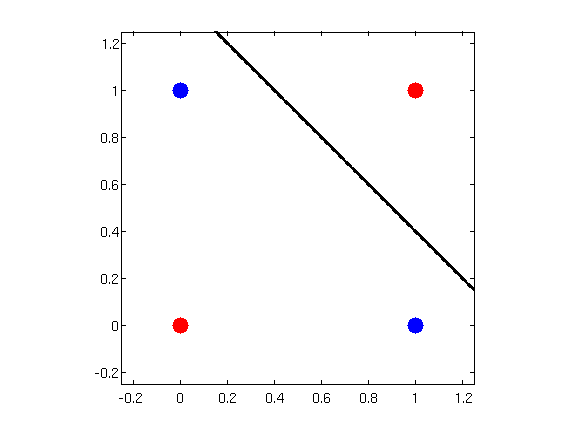## 1. 何为感知机?## 2. 感知机可以解决什么问题？

【例1】设有4个样本点，$$(0,0),(0,1),(1,0),(1,1)$$，根据$$OR$$的逻辑，必须至少有一个不为$$0$$才能判定为真，翻译成机器学习的表达即标签为$$(-1,1,1,1)$$，这里负数表示负样本，正数表示正样本。1. 代入$$(0,0)$$点，$$sign(wx + b) = sign(0 * 0 + 0 * 0 + 0*(-1)) = 0$$，无法判定是否为负类，错误。

• 更新权重

$$b = 0 - 1 * (1-0) * (-1)=1$$

$$w_1 = 0 - 1 * (1-0) * 0=0$$

$$w_2 = 0 - 1 * (1-0) * 0=0$$

2. 代入$$(0,1)$$点，$$sign(wx + b) = sign(0 * 0 + 0 * 1 +1 *(-1)) = -1$$，判定为负类，错误。

• 更新权重

$$b = 1 - 1 * (1 - (-1)) * (-1) = -1$$

$$w_1 = 0 - 1 * (1 - (-1))*0 = 0$$

$$w_2 = 0 - 1*(1-(-1))*1 = 2$$

3. 代入$$(1,0)$$点，$$sign(wx+b)=sign(0 * 1 + 2 * 0 + (-1) * (-1))= 1$$，判定为正类，正确。

4. 代入$$(1,1)$$点，$$sign(wx + b)=sign(0 * 1 + 2 * 1 + （-1）* -1)=3$$，判定为正类，正确。

5. 第二轮遍历，代入$$(0,0)$$$$sign(0*0 + 2*0 + （-1）*-1)=1$$，无法判定是否为负类，错误。

• 更新权重

$$b = -1 - 1*(1-(-1))*(-1)=1$$

$$w_1 = 0 - 1*(1-(-1))*0=0$$

$$w_2 = 2 - 1*(1-(-1))*0 = 2$$

6. 代入$$(0,1)$$$$sign(0 * 0 + 2 * 1 + 1 * (-1))=1$$，判定为正类，正确。

## 3. 感知机不能解决什么问题？

$$XOR$$又称为异或，它的逻辑如下：

0 0 0
0 1 1
1 0 1
1 1 0## 4. 感知机是如何工作的？

1. 选取初始值$$w_0, b_0$$
2. 在训练集中选取一个数据点$$(x_i,y_i)$$
3. 检查在这个样本点上，模型是否会错误分类，如果错误分类，则更新$$w_i,b_i$$
4. 回到第2步，直到整个训练集中没有误分类点

1. 初始值是随机给的，赋值为0是一个简便的做法，但通常设置为一个很小的随机值，后面会详细讨论
2. 每次训练其实只用到一个样本点，即使训练集中有很多数据点亦是如此。这和 $$w_ix_i$$这个表达式的计算方式有关，详见上面的数值例子。
3. 算法的重点在误分类这里，那么如何定义误分类就非常要紧了。
4. 算法是一个迭代的过程，且要满足所有样本点都分类正确才停机。反过来理解，就是感知机算法只能运用于完全线性可分的数据集。如果数据集是不能完全线性可分的，感知机永远不会停机，除非训练之前先设置好停机条件，常见的比如设置训练次数或者误差上界（一旦误差小于$\varepsilon$ 即可停机)。

## 5. 该如何定义错误？

1. 找到描述样本点到超平面距离的计算式
2. 正确分类的样本点的距离和丢弃不看
3. 定义误分类的距离和，对它求最小值

$$R^n$$空间中，任何一个样本点$$x_0$$到超平面的距离公式 $$\frac{1}{||w||}|w\cdot x + b|$$。对于误分类来说，$$y_i(w \cdot x + b) < 0$$，于是如果有一个误分类点$$x_0$$，那么它到超平面的距离可以写作 $$-y_i\frac{1}{||w||}|w\cdot x + b|$$。对于所有的误分类来说，自然可以写出下面的损失函数：$L(w,b) = -\sum\limits_{i\in E} y_i \frac{1}{||w||}|w\cdot x + b|$。这里添加负号的目的是使得损失函数的值恒为正数，这样对它求最小值可以使用凸优化工具。显然地，误分类样本点越少，$$L(w,b)$$越小；误分类点离超平面的距离越小，$$L(w,b)$$越小。

## 6. 知错后如何改错？

$\frac{\partial L}{\partial w} = - \sum\limits_{x_i \in E} y_i x_i$

$\frac{\partial L}{\partial b} = - \sum\limits_{x_i \in E} y_i$

## 7. $$Novikoff$$ 定理

(1) 存在满足条件$$||\hat{w}_{opt}|| = 1$$的超平面$$\hat{w}_{opt} \cdot \hat{x} = w_{opt} \cdot x + b_{opt}$$将训练数据集完全正确分开；且存在$$\gamma > 0$$，对所有的$$i = 1,2,...,N$$$y_i (\hat{w}_{opt} \cdot \hat{x}_i) = y_i (w_{opt} \cdot x_i + b_{opt}) \ge \gamma$

(2)令$$R = \max\limits_{1 \le i \le N} ||\hat{x}_i||$$，则感知机算法在训练数据集上的误分类次数$$k$$满足不等式 $k \le (\frac{R}{\gamma})^2$

(1) 由于数据是线性可分的，因此一定存在一个分割超平面$$S$$，不妨就取$$S$$的参数为$$\hat{w}_{opt}$$，显然对于超平面上的所有点$$x$$，都有$$\hat{w}_{opt} \cdot x = w_{opt} \cdot x + b_{opt} = 0$$，并满足$$||\hat{w}_{opt}||=1$$。而对于训练数据集中的样本点，由于现在都被正确分类了，所以有$$\hat{w}_{opt} \cdot x_i > 0$$，于是取 $$\gamma = \min\limits_{i} \{y_i(w_{opt} \cdot x_i + b_{opt} \}$$，这就得到了下界。

(2) 由 $$k \le (\frac{R}{\gamma})^2$$推出$$k \gamma \le \sqrt{k} R$$

• $$\hat{w}_{k} \cdot \hat{w}_{opt} = \hat{w}_{k-1} \cdot \hat{w}_{opt} + \eta y_i \hat{w}_{opt} \cdot \hat{x}_i \ge \hat{w}_{k-1} \cdot \hat{w}_{opt} + \eta \gamma$$ .

迭代$$k-1$$次立即得到 $$\hat{w}_{k} \cdot \hat{w}_{opt} \ge k\eta\gamma$$.

• $$\hat{w}_k$$两边平方，

$$||\hat{w}_k||^2 = ||\hat{w}_{k-1}||^2 + 2\eta y_i \hat{w}_{k-1} \cdot \hat{x}_{i} + \eta^2 ||\hat{x}_{i}||^2$$. 注意到中间这项是负数(因为这是误分类点)

立即得到 $$||\hat{w}_k||^2 \le ||\hat{w}_{k-1}||^2 + \eta^2 ||\hat{x}_{i}||^2 \le ||\hat{w}_{k-1}||^2 + \eta^2 R^2$$.

迭代$$k-1$$次后立即得到 $$||\hat{w}_k||^2 \le k \eta^2 R^2$$ .

$$k\eta\gamma \le \hat{w}_{k} \cdot \hat{w}_{opt} \le ||\hat{w}_{k}|| \cdot ||\hat{w}_{opt}|| \le \sqrt{k}\eta R$$. 再稍加变形就得到欲证的式子。